# Solution May 22, 2007

### Problem

Prove or disprove:

For any positive integer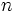$n\,$ there is a positive integer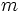$m\,$ such that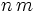$n\,m$ has only 0's and 7's as decimal digits.

### Solution

This is true.

Consider the sequence 7, 77, 777, etc, formally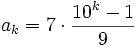$a_k = 7\cdot\frac{10^k-1}9$

modulo$n\,$. This is an infinite sequence but there are only finitely many (namely,$n\,$) residues modulo$n\,$ so let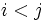$i be chosen such that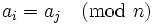$a_i = a_j \pmod n\,$.

Then, the difference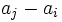$a_j - a_i\,$ is divisible by$n\,$. Its decimal digits form a sequence of 7s followed by 0s, so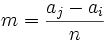$m=\frac{a_j-a_i}n$ satisfies all requirements of the problem.